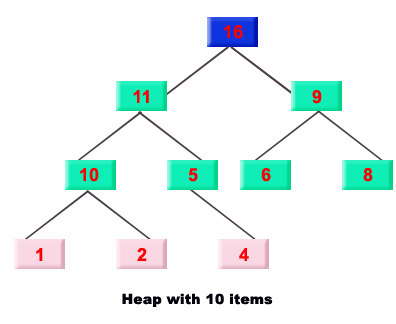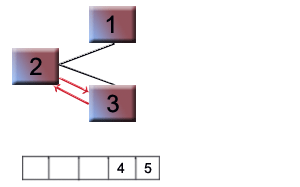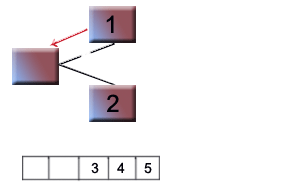# Heap Sort in Java

In this example we are going to sort integer values of an array using heap sort. There are two types of heap. First one is Max heap and second one is min heap. Max heap is a special type of binary tree .The roots of the max heap is greater than its c### Heap Sort in Java

In this example we are going to sort integer values of an array using heap sort. There are two types of heap. First one is Max heap and second one is min heap. Max heap is a special type of binary tree .The roots of the max heap is greater than its c# Heap Sort in Java

Introduction

In this example we are going to sort integer values of an array using heap sort.

There are two types of heap. First one is  Max heap and second one is min heap. Max heap is a special type of binary tree .The roots of  the max heap is greater than its child roots. Other heap is min heap it is also a special type of heap which has minimum root than his child. We can sort the array values using heap sorting algorithm. In this algorithm the heap build is used to rebuild the heap.
In this example we sorting all elements of an array. The complexity   of the heap sort is O(n.log(n)). Heap sort is slowest  but it is better option for large data sets.

The example of Max heap:Code description:
To sort a heap  Build Heap algorithm is used to build a heap out of the data set .Then remove the root element and replace the last element at the position of root node. Then rearrange the heap. Place the root node in an array. Follow these steps until all elements in heap is not replace into array. The values in array will be in sorted order.

Steps of heap sort algorithm:
1. Remove the parent root and replace it with the rightmost leaf.
2.Strore parent root in an array.
3. Re-establish the heap.
4. Repeat steps 1 and 3  until values in heap is not zero.

Working of heap sort
algorithm:
Input:1,3,5,4,2
Step1:
Buid Heap tree and an array of same size.Step2:
Remove largest root and  add largest root in array.Step3:
Replace last value (eg 2) at at root node position.Step4:
Swap 2 and 4Step5:
Swap 2 and 3.Step6:
Remove 4 and replace 2 at position of 4 and add 4 in arrayStep7:
Swap 2 and 3Step8:
Remove 3 ,add in array and replace 1 at position of 3.Step9:
Swap 2 and 1.Step10:
Remove 2 and it at root positionStep11:
Remove 1 and add in array.

Output:
Sorted array 1,2,3,4,5

The code of the program :

 ``` public class heap_Sort{   public static void main(String a[]){   int i;   int arr[] = {1,3,4,5,2};   System.out.println("\n  Heap Sort\n---------------\n");   System.out.println("\n  Unsorted Array\n\n");   for (i = 0; i < arr.length; i++)   System.out.print(" "+arr[i]);   for(i=arr.length; i>1; i--){   fnSortHeap(arr, i - 1);   }   System.out.println("\n  Sorted array\n---------------\n");   for (i = 0; i < arr.length; i++)   System.out.print(" "+arr[i]);   }   public static void fnSortHeap(int array[], int arr_ubound){   int i, o;   int lChild, rChild, mChild, root, temp;   root = (arr_ubound-1)/2;   for(o = root; o >= 0; o--){   for(i=root;i>=0;i--){   lChild = (2*i)+1;   rChild = (2*i)+2;   if((lChild <= arr_ubound) && (rChild <= arr_ubound)){   if(array[rChild] >= array[lChild])   mChild = rChild;   else   mChild = lChild;   }   else{   if(rChild > arr_ubound)   mChild = lChild;   else   mChild = rChild;   }   if(array[i] < array[mChild]){   temp = array[i];   array[i] = array[mChild];   array[mChild] = temp;   }   }   }   temp = array;   array = array[arr_ubound];   array[arr_ubound] = temp;   return;   } }```

Output of the example:

 `C:\array\sorting>Javac heap_Sort.java` `C:\array\sorting>java heap_Sort` ``` Heap Sort --------------- ``` ``` Unsorted Array ``` ``` 1 3 4 5 2 Sorted array ---------------``` ``` 1 2 3 4 5 C:\array\sorting>_```